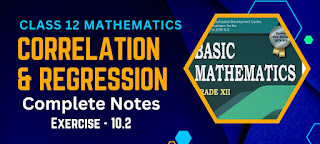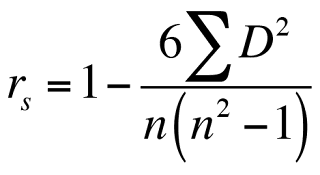# Class 12 Mathematics Correlation and Regression Notes | Exercise - 10.2We all are searching for the Notes of Correlation and Regression Class 12 Mathematics Solutions. It is very hard to find notes right now because the syllabus has been updated recently. It may be hard for other to provide notes for you but not for us.

We are here with the notes according to the syllabus of 2080. Here you'll get the complete notes of exercise 10.2 of correlation and regression chapter. If you are willing to complete your notes offline then you can download the full PDF of this exercise from the download link given below.

## Chapter - 10  Correlation and Regression

Correlation and regression is the 10th chapter of class 12 mathematics. This is the chapter which hasn't been replaced while updating the syllabus. However few new exercises has been added in the latest syllabus of 2080. New exercise has been added means we will be reading some new subtopics under this chapter.

In our previous post, we have briefly discussed about correlation, nature of correlation, methods of studying correlation, scatter diagram, Karl Pearson's correlation coefficient and some merits and limitations of Karl Pearson's correlation coefficient. So here we'll be discussing about some other topics.

### Rank Correlation

The degree of relationship between two variables with respect to their respective ranks is known as "Rank correlation coefficient". It is also known as Speraman's rank correlation coefficient.

Let R₁ and R₂ be the ranks of n individuals according to the characteristics say A and B respectively. If we assume that no two individuals have the same rank in a character then R, and R₂ take numerical values from 1 to n. Now, Spearman's rank correlation coefficient denoted by R is given by the formula:

R = 1 - (6Σd²)/{n(n² - 1)}
where d = R₁ - R₂, n = no. of pair of observations. The value of R also lies between -1 and +1.There are many other formulas used to calculate Spearman's rank correlation coefficient. Among them this is one. It is applicable when no. of pair of observations are given and when you have difference between the two ranks of each observations.

Exercise - 10.2

As mentioned earlier, this PDF only contains the notes of second exercise of correlation and regression chapter i.e exercise-10.2. If you want the notes of other exercises then you may find them above.

Note: Scroll the PDF to view all solutions

### Methods of calculating rank correlation coefficient

We obtain rank correlation coefficient under the following conditions:

• When actual ranks are given
• When ranks are not given

#### When actual ranks are given

When ranks are given the following steps are to be used for calculating the rank correlation coefficient.

• Find the difference between the ranks and denote it by d.
• Find d² and obtain Σd².
• Use the formula, R = 1 - (6Σd²)/{n(n² - 1)}.

Note: For correct ranking, Σd = 0.

#### When ranks are not given

If ranks be not given, we rank the given data by giving the highest value as 1 and next highest value 2 and so on. The same procedure is used for both variables under consideration. Rest procedure is same as in case (a).

Note: We can assign 1 to the lowest value and 2 to next lowest value and so on.

### Repeated ranks

If two or more variate values are equal then each equal value is given the common rank. In such cases, each individual is given an average rank. For example, if two individuals are ranked equal at 4th place, they are each given the rank, (4+5)÷2 = 4.5 and then the succeeding value the 6th rank and so on.

Some adjustments are to be made in the above Spearman's rank correlation formula for repeated ranks. In the above formula an extra factor, m(m² - 1)/12 is to be added to Σd² where m is the no. of times a variate values are repeated. This correlation factor is to be added for each repeated value in both series. After adjustment, Spearman's rank correlation coefficient R is:### Interpretation of Rank Correlation Coefficient

The interpretation of rank correlation coefficient is same as that of Karl Pearson's correlation coefficient. Its value R lies between -1 and 1 i.e. - 1 ≤ R ≤ 1. If R = 1, there is a perfect positive correlation between the two ranks of two judges. If R = 0, there is no relationship between the ranks of two judges.

### Merits and limitations of rank correlation

Merits:

• This method is easier compared to Karl Pearson's method.
• This is the only method of finding the relationship when ranks are given.
• This method is appropriate for qualitative characteristics such as intelligence, beauty etc.

Limitations:

• When the number of observations is greater than 30, the calculation will be tedious and consume a lot of time.
• It can not be used for bivariate frequency distribution.

### FAQs

What is rank correlation?

Rank correlation is a statistical technique used to measure the degree of association between two variables that are measured on an ordinal scale.

What is the difference between Spearman's rank correlation and Kendall's rank correlation?

The main difference between Spearman's rank correlation and Kendall's rank correlation is in how they handle tied ranks. Spearman's rank correlation is based on the difference between the ranks of the two variables, while Kendall's rank correlation is based on the number of concordant and discordant pairs of observations. Both coefficients range from -1 to 1, with higher values indicating a stronger correlation.

Can rank correlation be used to determine causality between two variables?

No, rank correlation cannot be used to determine causality between two variables. Correlation only measures the degree of association between two variables, but it does not imply causation. To establish causality, additional evidence and analyses are needed, such as experimental designs or causal inference methods.

Can rank correlation be used with continuous data?

Rank correlation is typically used with ordinal data, but it can also be used with continuous data that has been converted to ranks. However, using rank correlation with continuous data can result in a loss of information and reduced power compared to using linear correlation.

No Comment